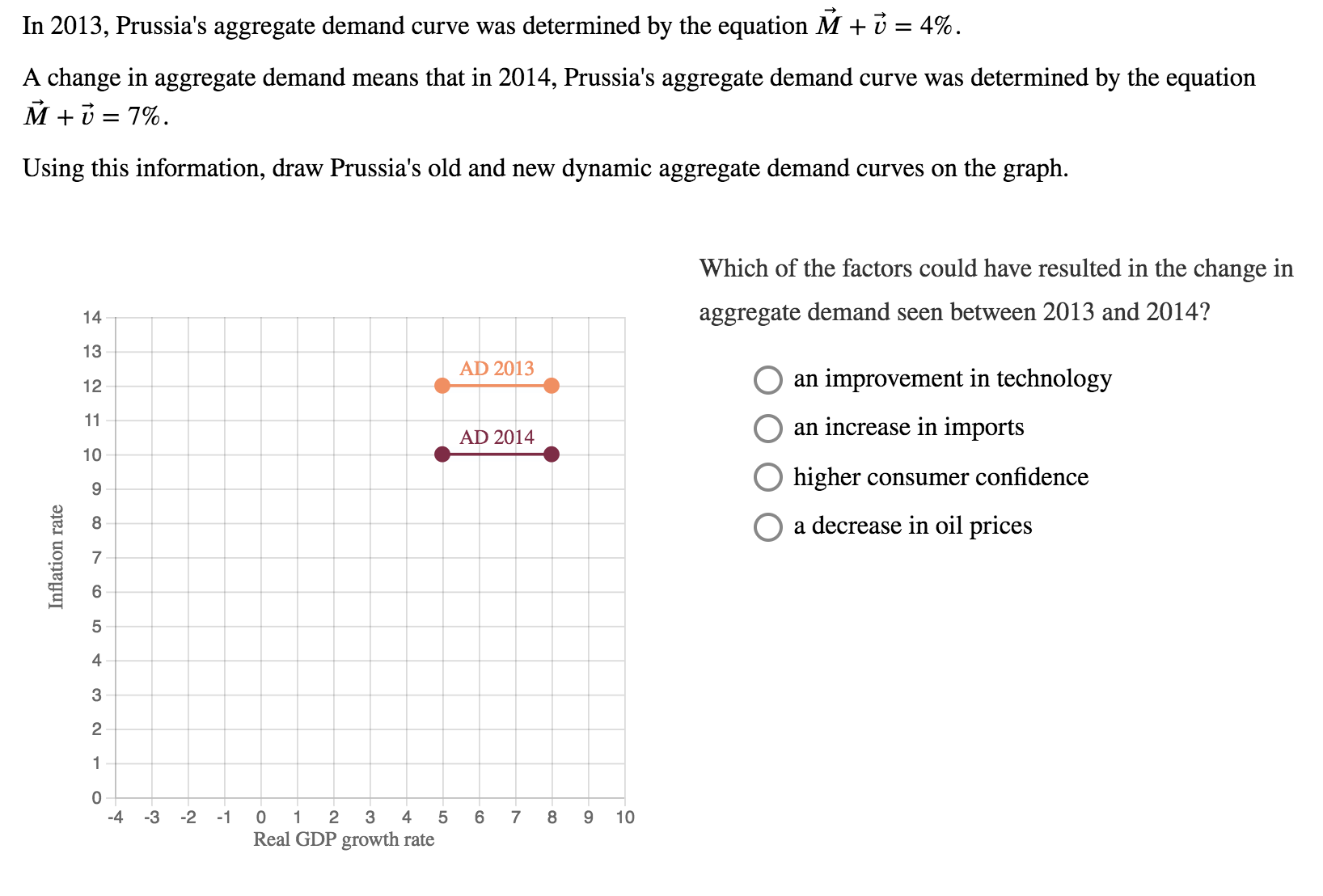# In 2013, Prussia's aggregate demand curve was determined by the equation M + 1-4%A change in aggregate demand means that in 2014, Prussia's aggregate demand curve was determined by the equationUsing this information, draw Prussia's old and new dynamic aggregate demand curves on the graphWhich of the factors could have resulted in the change irnaggregate demand seen between 2013 and 2014?13AD 2013an improvement in technologyO an increase in importsO higher consumer confidenceO a decrease in oil prices12AD 201410854324 -3 2 1 0 1 2 3 4 5 6 78 9 10Real GDP growth rate

Question
371 viewshelp_outlineImage TranscriptioncloseIn 2013, Prussia's aggregate demand curve was determined by the equation M + 1-4% A change in aggregate demand means that in 2014, Prussia's aggregate demand curve was determined by the equation Using this information, draw Prussia's old and new dynamic aggregate demand curves on the graph Which of the factors could have resulted in the change irn aggregate demand seen between 2013 and 2014? 13 AD 2013 an improvement in technology O an increase in imports O higher consumer confidence O a decrease in oil prices 12 AD 2014 10 8 5 4 3 2 4 -3 2 1 0 1 2 3 4 5 6 78 9 10 Real GDP growth rate fullscreen
check_circle

Step 1

The quantity theory of money:

The quantity theory of money equation can be written as follows:

Step 2

a.

2013:

According to the theory,

If M+V = 4%, then P+Y = 4%

Figure -1, shows the Prussia’s aggregate demand curve:

The combination point of real GDP and inflation is (-4%, 8%) and (4%, 0%)

Step 3

b.

2014:

According to the theory,

If M+V = 7%, then P+Y = 7%

Figure -2, shows the Prussia’s 2014 aggregate demand curve:

The...

### Want to see the full answer?

See Solution

#### Want to see this answer and more?

Solutions are written by subject experts who are available 24/7. Questions are typically answered within 1 hour.*

See Solution
*Response times may vary by subject and question.
Tagged in